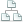Home >Tips >Excel 2016 from Scratch >Visualization J, pivot table and graph, DAVERAGE and DSUM calculationSite map

# An Excel tutorial by Peter KalmstromThe Excel function DAVERAGE calculates the average of the numbers in a column based on a given criteria, and in the same way the DSUM function gives the sum of the numbers in a column that meet a given criteria.

This demo in the Excel 2016 from Scratch series shows both calculation and visualization. Peter Kalmstrom explains how to calculate the average and sum of certain parameters, and then he presents the data in a pivot table and a pivot graph.

### Exercise

If you want to try the exercise yourself, please download the Excel file Peter uses in the demo.

### Content

This is what Peter shows in the demo below:
• How to format a range of cells as a table.
• How to use the function guide to create a formula that calculates the average of values in certain cells in a column using the DAVERAGE function.
• How to use the function guide to create a formula that calculates the sum of values in certain cells in a column using the DSUM function.
• How to create a pivot table to summarize data.
• How to create a view in a pivot table.
• How to insert a chart that visualizes the pivot table view.
Peter uses Excel 2016 for his demo, but the Excel visualization tools are the same for earlier versions of Excel.